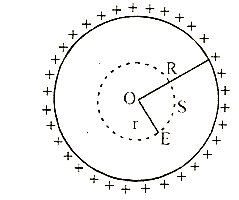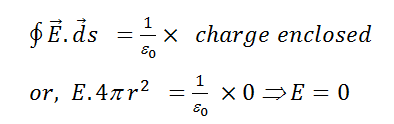# A hollow insulated conduction sphere is given a positive charge of 10 mC. What will be the electric field at the centre of the sphere if its radius is 2 metres?         (a)    Zero         (b)    5 uCm-2         (c)    20 uCm-2         (d)    8 uCm-2

9 years ago

(a)

Charge resides on the outer surface of a conducting hollow sphere of radius R. We consider a spherical surface of radius r < R. By Gauss''s theorem,i.e., electric field inside a hollow sphere is zero.

3 years ago
Field inside a conducting sphere is zero.
Formula of gausses law,
Φ=q/∈0
put q=0, final ans will be zero.
Hope it helps!